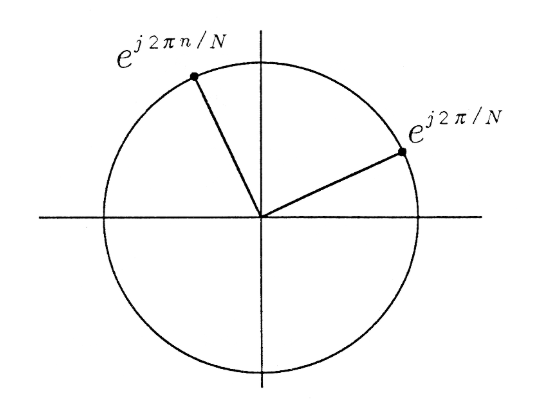Skip to main content

# 4.5: Roots of Unity and Related Topics

The complex number $$z=e^{j2π/N}$$ is illustrated in Figure $$\PageIndex{1}$$. It lies on the unit circle at angle $$θ=2π/N$$. When this number is raised to the nth power, the result is $$z^n=e^{j2πn/N}$$. This number is also illustrated in Figure $$\PageIndex{1}$$. When one of the complex numbers $$e^{j2πn/N}$$ is raised to the Nth power, the result is

$(e^{j2πn/N})^N=e^{j2πn}=1$We say that $$e^{j2πn/N} is one of the Nth roots of unity, meaning that \(e^{j2πn/N}$$ is one of the values of z for which

$z^N−1=0$

There are N such roots, namely,

$e^{j2πn/N},n=0,1,...,N−1$

As illustrated in the Figure $$\PageIndex{2}$$, the 12th roots of unity are uniformly distributed around the unit circle at angles $$2πn/12$$. The sum of all of the Nth roots of unity is zero:

$S_N=\sum_{n=0}^{N−1}e^{j2πn/N}=0$

This property, which is obvious from the Figure, is illustrated in Figure, where the partial sums $$S_k=\sum_{n=0}^{k−1} e^{j2πn/N}$$ are plotted for $$k=1,2,...,N$$.These partial sums will become important to us in our study of phasors and light diffraction in "Phasors" and in our discussion of filters in "Filtering".## Geometric Sum Formula

It is natural to ask whether there is an analytical expression for the partial sums of roots of unity:

$S_k=\sum_{n=0}^{k−1}e^{j2πn/N}$

We can imbed this question in the more general question, is there an analytical solution for the “geometric sum”

$S_k=\sum_{n=0}^{k−1}z^n?$

The answer is yes, and here is how we find it. If $$z=1$$, the answer is $$S_k=k$$. If $$z≠1$$, we can premultiply $$S_k$$ by $$z$$ and proceed as follows:

\begin{align*} zS_k &=\sum^{k−1}_{n=0}z^{n+1}=\sum^k_{m=1}z^m \\[4pt] &=\sum^{k−1}_{m=0}z^m+z^k−1 \\[4pt] &=S_k+z^k−1 \end{align*}

From this formula we solve for the geometric sum:

$S_k=\begin{matrix}\frac {1−z^k} {1−z} & z≠1\\k & z=1\end{matrix}$

This basic formula for the geometric sum Sk is used throughout electromagnetic theory and system theory to solve problems in antenna design and spectrum analysis. Never forget it.

Exercise $$\PageIndex{1}$$

Find formulas for $$S_k=\sum_{n=0}^{k−1}e^{jnθ}$$ and for $$S_k=\sum_{n=0}^{k−1}e^{j2π/Nn}$$.

Exercise $$\PageIndex{2}$$

Prove $$\sum_{n=0}^{N−1}e^{j2πn/N}=0$$.

Exercise $$\PageIndex{3}$$

Find formulas for the magnitude and phase of the partial sum $$S_k=\sum_{n=0}^{k−1}e^{j2πn/N}$$.

Exercise $$\PageIndex{4}$$

(MATLAB) Write a MATLAB program to compute and plot the partial sum $$S_k=\sum_{n=0}^{k−1}e^{j2πn/N}$$ for $$k=1,2,...,N$$. You should observe the last Figure.

Exercise $$\PageIndex{5}$$

Find all roots of the equation $$z^3+z^2+3z−15=0$$.

Exercise $$\PageIndex{6}$$

Find $$c$$ so that $$(1+j)$$ is a root of the equation $$z^{17}+2z^{15}−c=0$$.